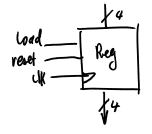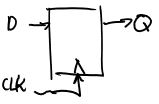# Sequential Logic

Updated 2019-04-03

Sequential logics are digital logic that contains memory, or states. The output depends on the input as well as whatever state a system may hold.

Flip flops (FF) or registers are a common type of sequential logic blocks. Here is an example of a 4-bit register with `load` and `reset` signals:Here is an example of a positive D-Flip-Flop. The FF holds the output and only changes to the input `D` when the input clock signal `clk` transitions on a positive edge (from low → high).Implemenation in Verilog:

``````module DFF(input D, input CLK, output Q);
always @(posedge CLK)
Q <= D;
endmodule
``````

Note that it may be ambiguous to differentiate between combinational logic vs. sequential logic when using `always` blocks. So to be safe, in SystemVerilog we use `always_ff` to explicitly indicate the logic we are writing is sequential logic.

``````module DFF(input D, input CLK, output Q);
always_ff @(posedge CLK)
Q <= D;
endmodule
``````

## Blocking & Non-Blocking

### Blocking Assignment

``````X = Y;
``````

Blocking assignments describes assignment that are immediate. Any subsequent reference to `X` uses the “new” values (value of `Y`).

### Non-Blocking Assignment

``````X <= Y;
``````

Non-blocking assignments describe assignments that are not immediate and happen at the end of the clock cycle. Thus, any subsequent reference to the “old” value before the update.

Ordering of assignment does not matter when using non-blocking assignments.